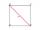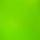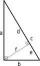# Two diagonals

The rhombus has a side length 12 cm and length of one diagonal 21 cm. What is the length of the second diagonal?

Result

u2 =  11.619 cm

#### Solution:Leave us a comment of example and its solution (i.e. if it is still somewhat unclear...):

Showing 0 comments:Be the first to comment!#### To solve this example are needed these knowledge from mathematics:

Pythagorean theorem is the base for the right triangle calculator.

## Next similar examples:

1. Diagonals in diamons/rhombusRhombus ABCD has side length AB = 4 cm and a length of one diagonal of 6.4 cm. Calculate the length of the other diagonal.
2. DiagonalsA diagonal of a rhombus is 20 cm long. If it's one side is 26 cm find the length of the other diagonal.
3. Rhombus IVCalculate the length of the diagonals of the rhombus, whose lengths are in the ratio 1: 2 and a rhombus side is 35 cm.
4. DiagonalsGiven a rhombus ABCD with a diagonalsl length of 8 cm and 12 cm. Calculate the side length and content of the rhombus.
5. SquareRectangular square has side lengths 183 and 244 meters. How many meters will measure the path that leads straight diagonally from one corner to the other?
6. SquareCalculate the side of a square with a diagonal measurement 10 cm.
7. Square2Side of the square is a = 6.2 cm, how long is its diagonal?
8. MedianIn the right triangle are sides a=41 dm b=42 dm. Calculate the length of the medians tc to the hypotenuse.
9. SatinSanusha buys a piece of satin 2.4 m wide. The diagonal length of the fabric is 4m. What is the length of the piece of satin?
10. CalculateCalculate the length of a side of the equilateral triangle with an area of 50cm2.
11. Height UTHow long is height in the equilateral triangle with a side b = 43?
12. Trio 2Decide whether trio of numbers is the side of a right triangle: 26,24,10.
13. ChordIn a circle with radius r=60 cm is chord 4× longer than its distance from the center. What is the length of the chord?
14. Triangle KLMIn the rectangular triangle KLM, where is hypotenuse m (sketch it!) find the length of the leg k and the height of triangle h if hypotenuse's segments are known mk = 5cm and ml = 15cm
15. Circle annulusThere are 2 concentric circles in the figure. Chord of larger circle 10 cm long is tangent to the smaller circle. What are does annulus have?
16. Completing squareSolve the quadratic equation: m2=4m+20 using completing the square method
17. The ditchDitch with cross section of an isosceles trapezoid with bases 2m 6m are deep 1.5m. How long is the slope of the ditch?